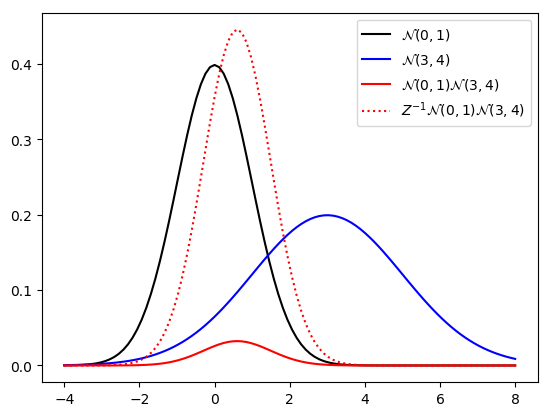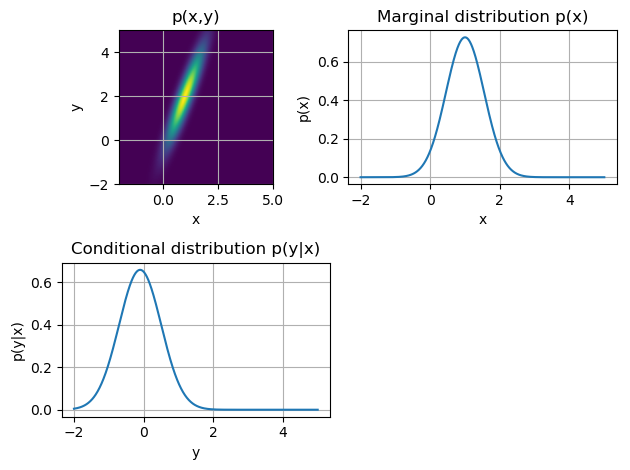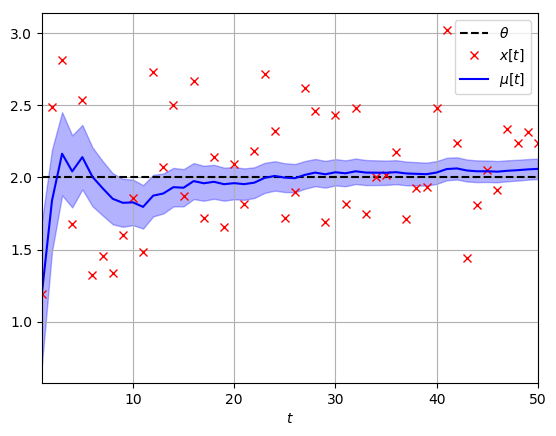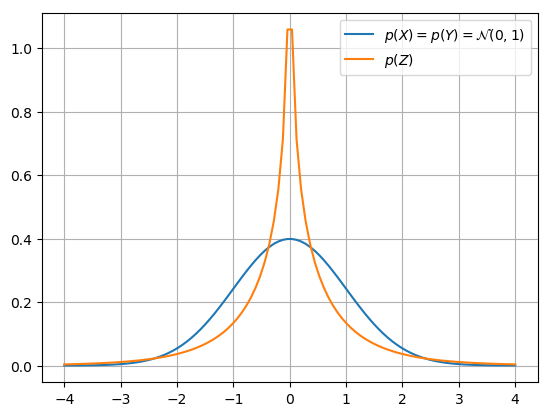# Working with Gaussians¶

### Sums and Transformations of Gaussian Variables¶

• The Gaussian distribution $$\mathcal{N}(x|\mu,\Sigma) = |2 \pi \Sigma |^{-\frac{1}{2}} \,\mathrm{exp}\left\{-\frac{1}{2}(x-\mu)^T \Sigma^{-1} (x-\mu) \right\}$$ for variable $x$ is completely specified by its mean $\mu$ and variance $\Sigma$.
• $\Lambda = \Sigma^{-1}$ is called the precision matrix.
• A linear transformation $z=Ax+b$ of a Gaussian variable $\mathcal{N}(x|\mu,\Sigma)$ is Gaussian distributed as
$$p(z) = \mathcal{N} \left(z \,|\, A\mu+b, A\Sigma A^T \right) \tag{SRG-4a}$$
• The sum of two independent Gaussian variables is also Gaussian distributed. Specifically, if $x \sim \mathcal{N} \left(x|\mu_x, \Sigma_x \right)$ and $y \sim \mathcal{N} \left(y|\mu_y, \Sigma_y \right)$, then the PDF for $z=x+y$ is given by \begin{align} p(z) &= \mathcal{N}(x\,|\,\mu_x,\Sigma_x) \ast \mathcal{N}(y\,|\,\mu_y,\Sigma_y) \notag\\ &= \mathcal{N} \left(z\,|\,\mu_x+\mu_y, \Sigma_x +\Sigma_y \right) \tag{SRG-8} \end{align}
• [Exercise]: Show that Eq.SRG-8 is really a special case of Eq.SRG-4a.
• The sum of two Gaussian distributions is NOT a Gaussian distribution. Why not?

### Example: Gaussian Signals in a Linear System¶• [Q.]: Given independent variables $x \sim \mathcal{N}(\mu_x,\sigma_y)$ and $y \sim \mathcal{N}(\mu_y,\sigma_y)$, what is the PDF for $z = A\cdot(x -y) + b$ ?
• [A.]: $z$ is also Gaussian with $$p_z(z) = \mathcal{N}(z|A(\mu_x-\mu_y)+b, \, A(\sigma_x \mathbf{+} \sigma_y)A^T)$$
• Think about the role of the Gaussian distribution for stochastic linear systems in relation to what sinusoidals mean for deterministic linear system analysis.

### Example: Bayesian Estimation of a Constant¶

• [Question] Estimate a constant $\theta$ from one 'noisy' measurement $x$ about that constant. Assume the following model specification (the tilde $\sim$ means: 'is distributed as'):
\begin{align*} x &= \theta + \epsilon \\ \theta &\sim \mathcal{N}(\mu_\theta,\sigma_\theta^2) \\ \epsilon &\sim \mathcal{N}(0,\sigma^2_{\epsilon}) \end{align*}

• 1. Model specification Note that you can rewrite these specifications in probabilistic notation as follows: \begin{align} p(x|\theta) &=\mathcal{N}(x|\theta,\sigma^2_{\epsilon}) \tag{likelihood}\\ p(\theta) &=\mathcal{N}(\theta|\mu_\theta,\sigma_\theta^2) \tag{prior} \end{align}
• 2. Inference for the posterior PDF $p(\theta|x)$ \begin{align*} p(\theta|x) &= \frac{p(x|\theta)p(\theta)}{p(x)} = \frac{p(x|\theta)p(\theta)} { \int p(x|\theta)p(\theta) \, \mathrm{d}\theta } \notag \\ &= \frac{1}{C} \,\mathcal{N}(x|\theta,\sigma^2_{\epsilon})\, \mathcal{N}(\theta|\mu_\theta,\sigma_\theta^2) \notag \\ &= \frac{1}{C_1} \mathrm{exp} \left\{ -\frac{(x-\theta)^2}{2\sigma^2_{\epsilon}} - \frac{(\theta-\mu_\theta)^2}{2\sigma_\theta^2} \right\} \notag \\ &= \frac{1}{C_1} \mathrm{exp} \left\{ \theta^2\left( -\frac{1}{2\sigma^2_{\epsilon}} - \frac{1}{2\sigma_\theta^2} \right) + \theta \left( \frac{x}{\sigma^2_{\epsilon}} + \frac{\mu_\theta}{\sigma_\theta^2} \right) + C_2 \right\} \notag \\ &= \frac{1}{C_1} \mathrm{exp} \left\{ -\frac{\sigma_\theta^2 + \sigma^2_{\epsilon}}{2\sigma_\theta^2 \sigma^2_{\epsilon}} \left( \theta - \frac{x\sigma_\theta^2 + \mu_\theta \sigma^2_{\epsilon}}{\sigma_\theta^2 + \sigma^2_{\epsilon}} \right)^2 + C_3 \right\} \end{align*} which we recognize as a Gaussian distribution.
• This computational 'trick' for multiplying two Gaussians is called completing the square. The procedure makes use of the equality $$ax^2+bx+c_1 = a\left(x+\frac{b}{2a}\right)^2+c_2$$
• Hence, it follows that the posterior for $\theta$ is
$$\begin{equation*} p(\theta|x) = \mathcal{N} (\theta |\, \mu_{\theta|x}, \sigma_{\theta|x}^2) \end{equation*}$$

where

\begin{align*} \frac{1}{\sigma_{\theta|x}^2} &= \frac{\sigma^2_{\epsilon} + \sigma_\theta^2}{\sigma^2_{\epsilon}\sigma_\theta^2} = \frac{1}{\sigma_\theta^2} + \frac{1}{\sigma^2_{\epsilon}}\\ \mu_{\theta|x} &= \sigma_{\theta|x}^2 \, \left( \frac{1}{\sigma^2_{\epsilon}}x + \frac{1}{\sigma_\theta^2} \mu_\theta \right) \end{align*}
• So, multiplication of two Gaussian distributions yields another (unnormalized) Gaussian with
• posterior precision equals sum of prior precisions
• posterior precision-weighted mean equals sum of prior precision-weighted means
• (This is worth remembering)

### CODE EXAMPLE¶

Let's plot the exact product of two Gaussian PDFs as well as the normalized product according to the above derivation.

In :
using PyPlot, Distributions
d1 = Normal(0, 1) # μ=0, σ^2=1
d2 = Normal(3, 2) # μ=3, σ^2=4

# Calculate the parameters of the product d1*d2
s2_prod = (d1.σ^-2 + d2.σ^-2)^-1
m_prod = s2_prod * ((d1.σ^-2)*d1.μ + (d2.σ^-2)*d2.μ)
d_prod = Normal(m_prod, sqrt(s2_prod)) # Note that we neglect the normalization constant.

# Plot stuff
x = range(-4, stop=8, length=100)
plot(x, pdf.(d1,x), "k")
plot(x, pdf.(d2,x), "b")
plot(x, pdf.(d1,x) .* pdf.(d2,x), "r-") # Plot the exact product
plot(x, pdf.(d_prod,x), "r:")          # Plot the normalized Gaussian product
legend([L"\mathcal{N}(0,1)",
L"\mathcal{N}(3,4)",
L"\mathcal{N}(0,1) \mathcal{N}(3,4)",
L"Z^{-1} \mathcal{N}(0,1) \mathcal{N}(3,4)"]);The solid and dotted red curves are identical up to a scaling factor $Z$.

### Multivariate Gaussian Multiplication¶

• In general, the multiplication of two multi-variate Gaussians yields an (unnormalized) Gaussian, see [SRG-6]: $$\begin{equation*} \mathcal{N}(x|\mu_a,\Sigma_a) \cdot \mathcal{N}(x|\mu_b,\Sigma_b) = \alpha \cdot \mathcal{N}(x|\mu_c,\Sigma_c) \end{equation*}$$ where \begin{align*} \Sigma_c^{-1} &= \Sigma_a^{-1} + \Sigma_b^{-1} \\ \Sigma_c^{-1} \mu_c &= \Sigma_a^{-1}\mu_a + \Sigma_b^{-1}\mu_b \end{align*} and normalization constant $\alpha = \mathcal{N}(\mu_a|\, \mu_b, \Sigma_a + \Sigma_b)$.
• If we define the precision as $\Lambda \equiv \Sigma^{-1}$, then we see that precisions add and precision-weighted means add too.
• As we just saw, great application to Bayesian inference!
$$\begin{equation*} \underbrace{\text{Gaussian}}_{\text{posterior}} \propto \underbrace{\text{Gaussian}}_{\text{likelihood}} \times \underbrace{\text{Gaussian}}_{\text{prior}} \end{equation*}$$

### Conditioning and Marginalization of a Gaussian¶

• Let $z = \begin{bmatrix} x \\ y \end{bmatrix}$ be jointly normal distributed as
\begin{align*} p(z) &= \mathcal{N}(z | \mu, \Sigma) =\mathcal{N} \left( \begin{bmatrix} x \\ y \end{bmatrix} \left| \begin{bmatrix} \mu_x \\ \mu_y \end{bmatrix}, \begin{bmatrix} \Sigma_x & \Sigma_{xy} \\ \Sigma_{yx} & \Sigma_y \end{bmatrix} \right. \right) \end{align*}
• Since covariance matrices are by definition symmetric, it follows that $\Sigma_x$ and $\Sigma_y$ are symmetric and $\Sigma_{xy} = \Sigma_{yx}^T$.
• Let's factorize $p(x,y)$ into $p(y|x)\, p(x)$ through conditioning and marginalization (proof in Bishop pp.87-89)
• Conditioning
\begin{align*} p(y|x) &= p(x,y)/p(x) \\ &= \mathcal{N}\left(y|\mu_y + \Sigma_{yx}\Sigma_x^{-1}(x-\mu_x),\, \Sigma_y - \Sigma_{yx}\Sigma_x^{-1}\Sigma_{xy} \right) \end{align*}
• Marginalization
$$p(x) = \int p(x,y)\,\mathrm{d}y = \mathcal{N}\left( x|\mu_x, \Sigma_x \right)$$
• Useful for applications to Bayesian inference in jointly Gaussian systems.

#### CODE EXAMPLE¶

Plot of the joint, marginal, and conditional distributions.

In :
using PyPlot, Distributions

μ = [1.0; 2.0]
Σ = [0.3 0.7;
0.7 2.0]
joint = MvNormal(μ,Σ)
marginal_x = Normal(μ, sqrt(Σ[1,1]))

#Plot p(x,y)
subplot(221)
x_range = y_range = range(-2,stop=5,length=1000)
joint_pdf = [ pdf(joint, [x_range[i];y_range[j]]) for  j=1:length(y_range), i=1:length(x_range)]
imshow(joint_pdf, origin="lower", extent=[x_range, x_range[end], y_range, y_range[end]])
grid(); xlabel("x"); ylabel("y"); title("p(x,y)"); tight_layout()

# Plot p(x)
subplot(222)
plot(range(-2,stop=5,length=1000), pdf.(marginal_x, range(-2,stop=5,length=1000)))
grid(); xlabel("x"); ylabel("p(x)"); title("Marginal distribution p(x)"); tight_layout()

# Plot p(y|x)
x = 0.1
conditional_y_m = μ+Σ[2,1]*inv(Σ[1,1])*(x-μ)
conditional_y_s2 = Σ[2,2] - Σ[2,1]*inv(Σ[1,1])*Σ[1,2]
conditional_y = Normal(conditional_y_m, sqrt.(conditional_y_s2))
subplot(223)
plot(range(-2,stop=5,length=1000), pdf.(conditional_y, range(-2,stop=5,length=1000)))
grid(); xlabel("y"); ylabel("p(y|x)"); title("Conditional distribution p(y|x)"); tight_layout()As is clear from the plots, the conditional distribution is a renormalized slice from the joint distribution.

### Example: Conditioning of Gaussian¶

• Consider (again) the system
\begin{align*} x &= \theta + \epsilon \\ \theta &\sim \mathcal{N}(\theta|\mu_\theta,\sigma_\theta^2) \\ \epsilon &\sim \mathcal{N}(\epsilon|0,\sigma^2_{\epsilon}) \end{align*}
• This system is equivalent to (derive this!)
$$p(x,\theta|\,\mu,\sigma) = \mathcal{N} \left( \begin{bmatrix} x\\ \theta \end{bmatrix} \left| \begin{bmatrix} \mu_\theta\\ \mu_\theta\end{bmatrix}, \begin{bmatrix} \sigma_\theta^2+\sigma_{\epsilon}^2 & \sigma_\theta^2\\ \sigma_\theta^2 &\sigma_\theta^2 \end{bmatrix} \right. \right)$$
• Direct substitution of the rule for Gaussian conditioning leads to (derive this yourself!) \begin{align*} p(\theta|x) &= \mathcal{N} \left( \theta\,|\,\mu_{\theta|x}, \sigma_{\theta|x}^2 \right)\,, \quad \text{with} \\ K &= \frac{\sigma_\theta^2}{\sigma_\theta^2+\sigma_{\epsilon}^2} \qquad \text{(K is called: Kalman gain)}\\ \mu_{\theta|x} &= \mu_\theta + K \cdot (x-\mu_\theta)\\ \sigma_{\theta|x}^2 &= \left( 1-K \right) \sigma_\theta^2 \end{align*}

$\longrightarrow$ Moral: For jointly Gaussian systems, we can do inference simply in one step by using the formulas for conditioning and marginalization.

### Application: Recursive Bayesian Estimation¶

Now consider the signal $x_t=\theta+\epsilon_t$, where $D_t= \left\{x_1,\ldots,x_t\right\}$ is observed sequentially (over time).

[Question]

• Derive a recursive algorithm for $p(\theta|D_t)$, i.e., an update rule for (posterior) $p(\theta|D_t)$ based on (prior) $p(\theta|D_{t-1})$ and (new observation) $x_t$.

• Let's define the estimate after $t$ observations (i.e., our solution) as $p(\theta|D_t) = \mathcal{N}(\theta\,|\,\mu_t,\sigma_t^2)$.
• Model specification. We define the joint distribution for $\theta$ and $x_t$, given background $D_{t-1}$, by \begin{align*} p(x_t,\theta \,|\, D_{t-1}) &= p(x_t|\theta) \, p(\theta|D_{t-1}) \\ &= \underbrace{\mathcal{N}(x_t\,|\, \theta,\sigma^2_{\epsilon})}_{\text{likelihood}} \, \underbrace{\mathcal{N}(\theta\,|\,\mu_{t-1},\sigma_{t-1}^2)}_{\text{prior}} \end{align*}
• Inference. Use Bayes rule, \begin{align*} p(\theta|D_t) &\propto p(x_t|\theta) \, p(\theta|D_{t-1}) \\ &= \mathcal{N}(x_t|\theta,\sigma^2_{\epsilon}) \, \mathcal{N}(\theta\,|\,\mu_{t-1},\sigma_{t-1}^2) \\ &= \mathcal{N}(\theta|x_t,\sigma^2_{\epsilon}) \, \mathcal{N}(\theta\,|\,\mu_{t-1},\sigma_{t-1}^2) \\ &= \mathcal{N}(\theta|\mu_t,\sigma_t^2) \end{align*} with \begin{align*} K_t &= \frac{\sigma_{t-1}^2}{\sigma_{t-1}^2+\sigma_{\epsilon}^2} \qquad \text{(Kalman gain)}\\ \mu_t &= \mu_{t-1} + K_t \cdot (x_t-\mu_{t-1})\\ \sigma_t^2 &= \left( 1-K_t \right) \sigma_{t-1}^2 \end{align*} (as before, we used the formulas for conditioning in a multivariate Gaussian system).
• This linear sequential estimator of mean and variance in Gaussian observations is called a Kalman Filter.
• Note that the uncertainty about $\theta$ decreases over time (since $0<(1-K_t)<1$). This makes sense: since we assume that the statistics of the system do not change (stationarity), each new sample provides new information.
• Recursive Bayesian estimation is the basis for adaptive signal processing algorithms such as Least Mean Squares (LMS) and Recursive Least Squares (RLS).

#### CODE EXAMPLE¶

Let's implement the Kalman filter described above. We'll use it to recursively estimate the value of $\theta$ based on noisy observations.

In :
using PyPlot

N = 50                         # Number of observations
θ = 2.0                        # True value of the variable we want to estimate
σ_ϵ2 = 0.25                    # Observation noise variance
x = sqrt(σ_ϵ2) * randn(N) .+ θ # Generate N noisy observations of θ

t = 0
μ = fill!(Vector{Float64}(undef,N), NaN)    # Means of p(θ|D) over time
σ_μ2 = fill!(Vector{Float64}(undef,N), NaN) # Variances of p(θ|D) over time

function performKalmanStep()
# Perform a Kalman filter step, update t, μ, σ_μ2
global t += 1
if t>1 # Use posterior from prev. step as prior
K = σ_μ2[t-1] / (σ_ϵ2 + σ_μ2[t-1]) # Kalman gain
μ[t] = μ[t-1] + K*(x[t] - μ[t-1])  # Update mean using (1)
σ_μ2[t] = σ_μ2[t-1] * (1.0-K)      # Update variance using (2)
elseif t==1 # Use prior
# Prior p(θ) = N(0,1000)
K = 1000.0 / (σ_ϵ2 + 1000.0) # Kalman gain
μ[t] = 0 + K*(x[t] - 0)      # Update mean using (1)
σ_μ2[t] = 1000 * (1.0-K)     # Update variance using (2)
end
end

while t<N
performKalmanStep()
end

# Plot the 'true' value of θ, noisy observations x, and the recursively updated posterior p(θ|D)
t = collect(1:N)
plot(t, θ*ones(N), "k--")
plot(t, x, "rx")
plot(t, μ, "b-")
fill_between(t, μ-sqrt.(σ_μ2), μ+sqrt.(σ_μ2), color="b", alpha=0.3)
legend([L"\theta", L"x[t]", L"\mu[t]"])
xlim((1, N)); xlabel(L"t"); grid()The shaded area represents 2 standard deviations of posterior $p(\theta|D)$. The variance of the posterior is guaranteed to decrease monotonically for the standard Kalman filter.

### Product of Normally Distributed Variables¶

• (We've seen that) the sum of two Gausssian distributed variables is also Gaussian distributed.
• Has the product of two Gaussian distributed variables also a Gaussian distribution?
• No! In general this is a difficult computation. As an example, let's compute $p(z)$ for $Z=XY$ for the special case that $X\sim \mathcal{N}(0,1)$ and $Y\sim \mathcal{N}(0,1)$. \begin{align*} p(z) &= \int_{X,Y} p(z|x,y)\,p(x,y)\,\mathrm{d}x\mathrm{d}y \\ &= \frac{1}{2 \pi}\int \delta(z-xy) \, e^{-(x^2+y^2)/2} \, \mathrm{d}x\mathrm{d}y \\ &= \frac{1}{\pi} \int_0^\infty \frac{1}{x} e^{-(x^2+z^2/x^2)/2} \, \mathrm{d}x \\ &= \frac{1}{\pi} \mathrm{K}_0( \lvert z\rvert )\,. \end{align*} where $\mathrm{K}_n(z)$ is a modified Bessel function of the second kind.

#### CODE EXAMPLE¶

We plot $p(Z)$ to give an idea of what this distribution looks like.

In :
using PyPlot, Distributions, SpecialFunctions
X = Normal(0,1)
pdf_product_std_normals(z::Vector) = (besselk.(0, abs.(z))./π)
range1 = collect(range(-4,stop=4,length=100))
plot(range1, pdf.(X, range1))
plot(range1, pdf_product_std_normals(range1))
legend([L"p(X)=p(Y)=\mathcal{N}(0,1)", L"p(Z)"]); grid()### Review Gaussians¶

The success of Gaussian distributions in probabilistic modeling is large due to the following properties:

• A linear transformation of a Gaussian distributed variable is also Gaussian distributed
• The convolution of two Gaussian functions is another Gaussian function (use in sum of 2 variables)
• The product of two Gaussian functions is another Gaussian function (use in Bayes rule).
• Conditioning and marginalization of multivariate Gaussian distributions produce Gaussians again (use in working with observations and when doing Bayesian predictions)
• The Gaussian PDF has higher entropy than any other with the same variance. (Not discussed in this course).

• Any smooth function with single rounded maximum, if raised to higher and higher powers, goes into a Gaussian function. (Not discussed).

### Some Useful Matrix Calculus¶

Aside from working with Gaussians, it will be helpful for the next lessons to be familiar with some matrix calculus. We shortly recapitulate used formulas here.

• We define the gradient of a scalar function $f(A)$ w.r.t. an $n \times k$ matrix $A$ as $$\nabla_A f \triangleq \begin{bmatrix} \frac{\partial{f}}{\partial a_{11}} & \frac{\partial{f}}{\partial a_{12}} & \cdots & \frac{\partial{f}}{\partial a_{1k}}\\ \frac{\partial{f}}{\partial a_{21}} & \frac{\partial{f}}{\partial a_{22}} & \cdots & \frac{\partial{f}}{\partial a_{2k}}\\ \vdots & \vdots & \cdots & \vdots\\ \frac{\partial{f}}{\partial a_{n1}} & \frac{\partial{f}}{\partial a_{n2}} & \cdots & \frac{\partial{f}}{\partial a_{nk}} \end{bmatrix}$$
• The following formulas are useful (see Bishop App.-C) \begin{align*} |A^{-1}|&=|A|^{-1} \tag{B-C.4} \\ \nabla_A \log |A| &= (A^{T})^{-1} = (A^{-1})^T \tag{B-C.28} \\ \mathrm{Tr}[ABC]&= \mathrm{Tr}[CAB] = \mathrm{Tr}[BCA] \tag{B-C.9} \\ \nabla_A \mathrm{Tr}[AB] &=\nabla_A \mathrm{Tr}[BA]= B^T \tag{B-C.25} \\ \nabla_A \mathrm{Tr}[ABA^T] &= A(B+B^T) \tag{B-C.27}\\ \nabla_x x^TAx &= (A+A^T)x \tag{from B-C.27}\\ \nabla_X a^TXb &= \nabla_X \mathrm{Tr}[ba^TX] = ab^T \notag \end{align*}

### What's Next?¶

• We discussed how Bayesian probability theory provides an integrated framework for making predictions based on observed data.
• The process involves model specification (your main task!), inference and actual model-based prediction.
• The latter two tasks are only difficult because of computational issues.
• Maximum likelihood was introduced as a computationally simpler approximation to the Bayesian approach.
• In particular under some linear Gaussian assumptions, a few interesting models can be designed.
• The rest of this course (part-1) concerns introduction to these Linear Gaussian models.
In :
open("../../styles/aipstyle.html") do f